Courses
Courses for Kids
Free study material
Free LIVE classes
More

# Multiplication for KidsLIVE
Join Vedantu’s FREE Mastercalss

## Introduction to Multiplication

In math, multiplication is the method of finding the product of two or more numbers. Multiplication is one of the basic and most used mathematical operations. It is a fundamental operation in mathematics that is frequently utilized in everyday life. Generally, it is shown by writing the symbol '×' or '*' in between the two assigned numbers. When we need to combine groups of similar sizes, we utilize multiplication. In this article, we will learn about multiplication and will also see the multiplication problems.

## What is Multiplication?

Multiplication for class 3 is just multiplying two natural or whole numbers or integers together to obtain their product. '×' is used to denote the multiplication of two quantities. It can be stated as a method of adding the number repeatedly to acquire their product. For example: If we have to get the product of $2 \times 2$. So for it, we can add 2 + 2 to get the sum and product as 4.

## Parts of Multiplication

The multiplication formula is expressed as, Multiplicand $\times$ Multiplier $=$ Product; where:

• Multiplicand: The first number (factor).

• Multiplier: The second number (factor).

• Product: The final result after multiplying the multiplicand and multiplier.

Let us understand the multiplication parts with the help of the following expression.

35 (multiplicand) $\times 2$ (multiplier) $=70$ (product)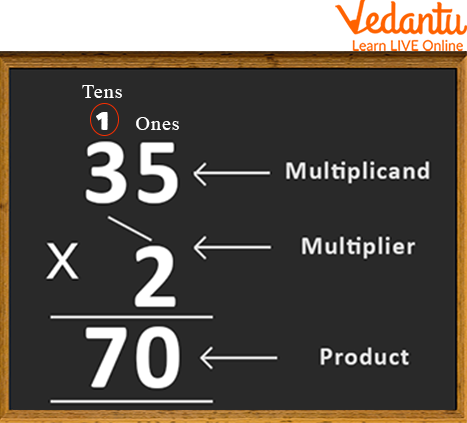Multiplication Parts

## Steps to Solve Multiplication Sums for Class 3

First, write the given multiplicand and multiplier in the column according to their place values, then put the cross sign before the multiplier. When we multiply two numbers, we always start multiplication from the left-hand side and then move to the right side.

For example (i) 35 × 2

Step 1: Write the provided numbers in columns

Step 2: Multiplying the multiplier with one place of the multiplicand, i.e. 5 × 2

Step 3: Then, multiply the tens place of multiplicand with the one's place of multiplier and add the carry to the product obtained.

Step 4: Thus, we get the required result, 35 × 2 = 70

## Multiplication Facts for Class 3

1. The two quantities being multiplied by each other are called factors.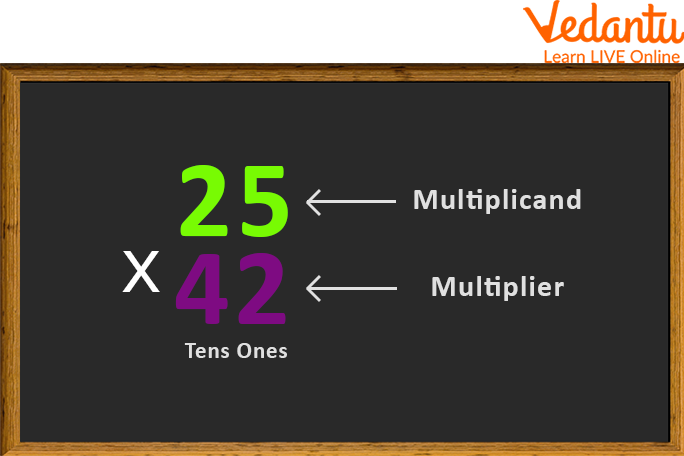Numbers used in Multiplication

1. Multiplication uses a specific property called a distributive property.

2. Any quantity multiplied by 1 produces the number itself as a result. i.e. $15 \times 1$ = 15

3. Any number multiplied by 0 produces the result of 0. i.e. $15 \times 0$ = 0

## Solved Examples

Q 1. 45 × 6

Ans: Align the given numbers in columns to apply the multiplication rule

• Multiplying the one's place of two numbers, i.e. 5 and 6, and writing the result in one's place

• Then, multiply the tens place of the multiplicand, say 4, with one's place of the multiplier, say 6 and add the carry, 3. Finally, write the result on the tens place

Thus, the product of 45 × 6 is 270.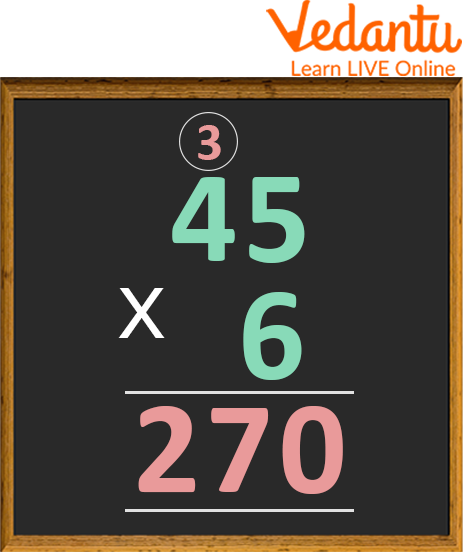Solved Example

Q 2. 65 × 31

Ans: Write in columns both the given numbers. Multiplying the one's place of two numbers, i.e. 5 and 1, and writing the result in one's place

• Then, multiply the tens place of the multiplicand, say 6, with one's place of the multiplier, say 1 and write the result on the tens place.

• Multiplying the tens place of multiplier with the one's place of multiplicand i.e. 5 and 3, and writing the product so obtained from tens place, leaving one’s place empty

• Now, multiply the tens place of multiplicand and multiplier together i.e. 6 and 3, and add one carry to the obtained product. Write the product in the tens place.

• Adding the two partial products obtained to get the final result, i.e. 65 + 1950 = 2015.

Hence, the required product of 65 × 31 is 2015.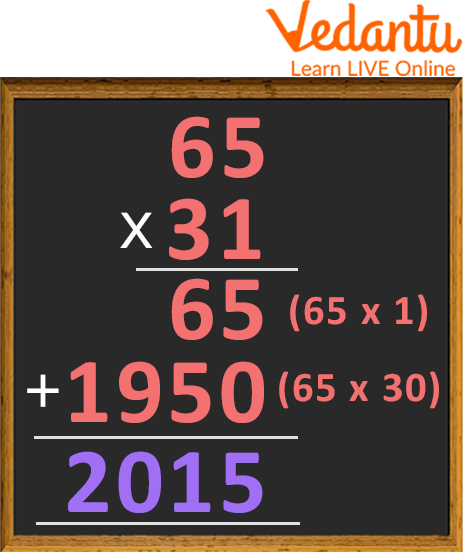Multiplication of Two-digit Numbers

## Multiplication Worksheet

Several multiplication sheets for class 3 are given below: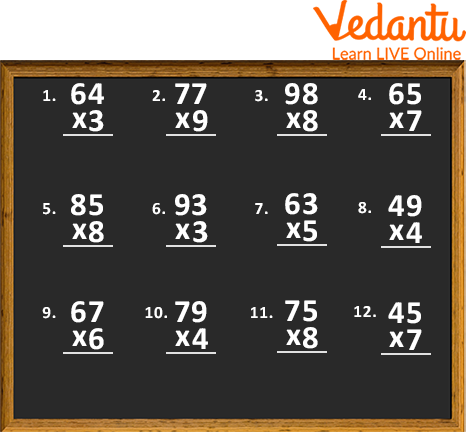Worksheet 1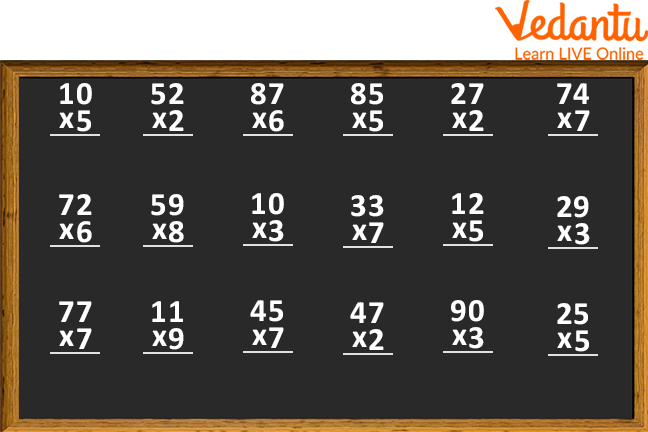Worksheet 2

## Practice Problems

Some multiplication problems to be solved on your own are given under:

Q 1. 59 × 3

Ans: 177

Q 2. 54 × 11

Ans: 594

Q 3. 29 × 8

Ans: 232

Q 4. 34 × 10
Ans: 340

Q 5. 90 × 32

Ans: 2,880

Q 6. 89 × 0

Ans: 0

## Summary

To sum up here with the concept of Multiplication of Class 3. The main motive of this article is to teach the students the easiest way to multiply any two given numbers. The steps involved in multiplication sums for class 3 are explained in depth in this article using the solved examples and images. Various multiplication problems and worksheets are also specified to strengthen the basic concept of multiplication in children's minds. Hoping the article helped you understand the concept of multiplication and you enjoyed reading it.

Last updated date: 20th Sep 2023
Total views: 94.5k
Views today: 0.94k

## FAQs on Multiplication for Kids

1. Name several properties of multiplication.

Some properties of multiplication include:

• Closure Property

• Commutative Property

• Distributive Property

• Associative Property

• Multiplicative Identity

• Multiplicative Inverse

2. Can a number be multiplied by itself?

Yes, a number can be multiplied by itself. It will produce the result in the square of the given number.

e.g. 2 × 2 = 4

where 4 is the square of 2, which is the given number.

3. How do you solve 3 digits multiplication problems?

3 digit multiplication problems are solved in the same way as two-digit multiplication problems. The only difference is in the multiplication of the digit at the hundredth place. Just multiply the multiplier with the digit at the hundredth place of the multiplicand, write the result obtained by leaving two places from the right, and then add all the partial products together to reach the final solution.